# Fourier Transform¶

The Fourier transform is: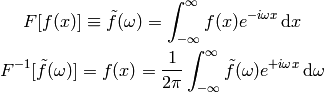To show that it works:# Laplace Transform¶

Laplace transform of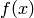is: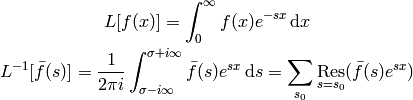The contour integration is over the vertical line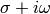and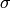is chosen large enough so that all residues are to the left of the line (that’s because the Laplace transform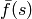is only defined for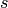larger than the residues, so we have to integrate in this range as well). It can be shown that the integral over the left semicircle goes to zero: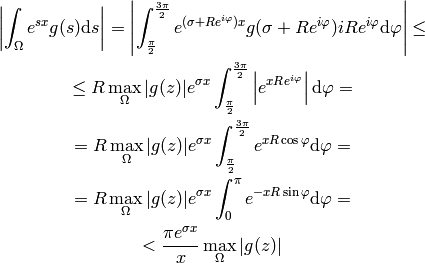so the complex integral is equal to the sum of all residues of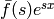in the complex plane.

To show that it works: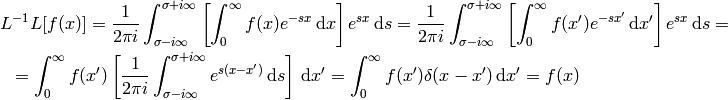where we used: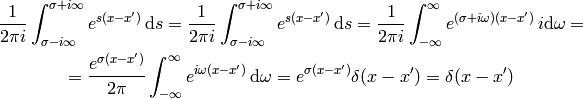and it can be derived from the Fourier transform by transforming a function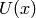: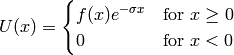and making a substitution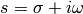: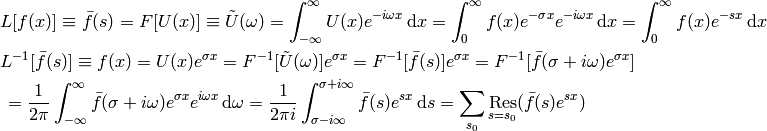Where the bar (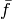) means the Laplace transform and tilde (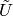) means the Fourier transform.